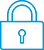cancel
Showing results for
Search instead for
Did you mean:It has been a while since anyone has replied. Simply ask a new question if you would like to start the discussion again.Level 1
5 2 0 1
Message 1 of 3
684
Flag Post

Solved!

# Issues with calculating y^xHP Recommended
HP 10bII+ Financial Calculator

This is my second post on trying to understand how this thing works. In working with the bond valuation formula

= Coupon * [(1-(1+i)^-n)/i]+M(1+i)^-n

I am doing each step of this equation separately because I dont know any other way for now, and my problem is when I get to the power functions.

In Excel I get these values

1.06^3 = 1.1910

1.06^-3 = 0.8396

In the calculator I get

1.06^3 = 1.1910

1.06^-3 = -1.9400 OR 0.8931

To get the first result my input is simply 1.06, red shift, y^x, -3

To get the second result my input is 1.06, red shift, y^x, red shift, open parenthesis, -3, red shift, close parenthesis

However, neither one of them seems correct based on the results I get on excel; so the question is, what am I doing wrong? For this particular formula it seems I will have to elevate numbers to the negative power pretty often so I really need to understand how this works.

As always, thank you all for your help in understnading this.

Cheers.

Tags (1)
2 REPLIES 2Level 9
971 954 119 186
Message 2 of 3
Flag PostHP Recommended

Hi,

I think you are using the normal minus key for the negative 3. You should use the +/- key after typing the 3, i.e.:

1.06

Y^x

3

+/-

=

and you should get 0.8396..

-Bart
_________________________________________________________
calculator enthusiast
Tags (1)Level 1
5 2 0 1
Message 3 of 3
Flag PostHP Recommended

That's it! Thank you so much!

† The opinions expressed above are the personal opinions of the authors, not of HP. By using this site, you accept the Terms of Use and Rules of Participation